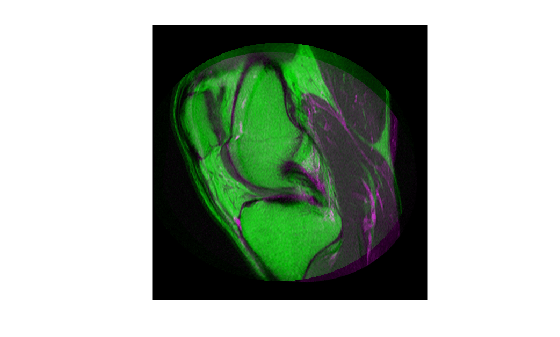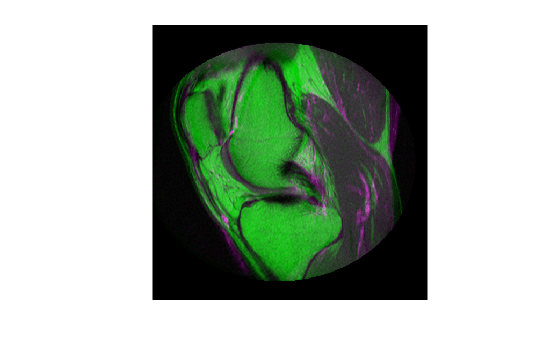Main Content

# MattesMutualInformation

Mattes mutual information metric configuration

## Description

A `MattesMutualInformation` object describes a mutual information metric configuration that you pass to the function `imregister` to solve image registration problems.

## Creation

You can create a `MattesMutualInformation` object using the following methods:

• `imregconfig` — Returns a `MattesMutualInformation` object paired with an appropriate optimizer for registering multimodal images

• Entering

`metric = registration.metric.MattesMutualInformation;`
on the command line creates a `MattesMutualInformation` object with default settings

## Properties

expand all

Number of spatial samples used to compute the mutual information metric, specified as a positive integer scalar. `NumberOfSpatialSamples` defines the number of random pixels `imregister` uses to compute the metric. Your registration results are more reproducible (at the cost of performance) as you increase this value. `imregister` only uses `NumberOfSpatialSamples` when `UseAllPixels = 0` (`false`).

Data Types: `double` | `single` | `uint8` | `uint16` | `uint32` | `uint64` | `int8` | `int16` | `int32` | `int64`

Number of histogram bins used to compute the mutual information metric, specified as a positive integer scalar. `NumberOfHistogramBins` defines the number of bins `imregister` uses to compute the joint distribution histogram. The minimum value is `5`.

Data Types: `double` | `single` | `uint8` | `uint16` | `uint32` | `uint64` | `int8` | `int16` | `int32` | `int64`

Option to compute the metric using all pixels in the overlap region of the images when computing the mutual information metric, specified as a logical scalar.

You can achieve significantly better performance if you set this property to `0` (`false`). When `UseAllPixels = 0`, the `NumberOfSpatialSamples` property controls the number of random pixel locations that `imregister` uses to compute the metric. The results of your registration might not be reproducible when `UseAllPixels = 0`. This is because `imregister` selects a random subset of pixels from the images to compute the metric.

## Examples

collapse all

Create a `MattesMutualInformation` object and use it to register two MRI images of a knee that were obtained using different protocols.

Read the images into the workspace. The images are multimodal because they have different brightness and contrast.

```fixed = dicomread('knee1.dcm'); moving = dicomread('knee2.dcm');```

View the misaligned images.

```figure imshowpair(fixed, moving,'Scaling','joint');```Create the optimizer configuration object suitable for registering multimodal images.

`optimizer = registration.optimizer.OnePlusOneEvolutionary;`

Create the metric configuration object suitable for registering multimodal images.

`metric = registration.metric.MattesMutualInformation`
```metric = registration.metric.MattesMutualInformation Properties: NumberOfSpatialSamples: 500 NumberOfHistogramBins: 50 UseAllPixels: 1 ```

Tune the properties of the optimizer so that the problem will converge on a global maxima. Increase the number of iterations the optimizer will use to solve the problem.

```optimizer.InitialRadius = 0.009; optimizer.Epsilon = 1.5e-4; optimizer.GrowthFactor = 1.01; optimizer.MaximumIterations = 300;```

Perform the registration.

`movingRegistered = imregister(moving,fixed,'affine',optimizer,metric);`

View the registered images.

```figure imshowpair(fixed, movingRegistered,'Scaling','joint');```## Tips

• Larger values of mutual information correspond to better registration results. You can examine the computed values of Mattes mutual information if you enable `'DisplayOptimization'` when you call `imregister`, for example:

`movingRegistered = imregister(moving,fixed,'rigid',optimizer,metric,'DisplayOptimization',true);`

## Algorithms

Mutual information metrics are information theoretic techniques for measuring how related two variables are. These algorithms use the joint probability distribution of a sampling of pixels from two images to measure the certainty that the values of one set of pixels map to similar values in the other image. This information is a quantitative measure of how similar the images are. High mutual information implies a large reduction in the uncertainty (entropy) between the two distributions, signaling that the images are likely better aligned.

The Mattes mutual information algorithm uses a single set of pixel locations for the duration of the optimization, instead of drawing a new set at each iteration. The number of samples used to compute the probability density estimates and the number of bins used to compute the entropy are both user selectable. The marginal and joint probability density function is evaluated at the uniformly spaced bins using the samples. Entropy values are computed by summing over the bins. Zero-order and third-order B-spline kernels are used to compute the probability density functions of the fixed and moving images, respectively .

 Rahunathan, Smriti, D. Stredney, P. Schmalbrock, and B.D. Clymer. Image Registration Using Rigid Registration and Maximization of Mutual Information. Poster presented at: MMVR13. The 13th Annual Medicine Meets Virtual Reality Conference; 2005 January 26–29; Long Beach, CA.

 D. Mattes, D.R. Haynor, H. Vesselle, T. Lewellen, and W. Eubank. "Non-rigid multimodality image registration." (Proceedings paper).Medical Imaging 2001: Image Processing. SPIE Publications, 3 July 2001. pp. 1609–1620.

Download ebook